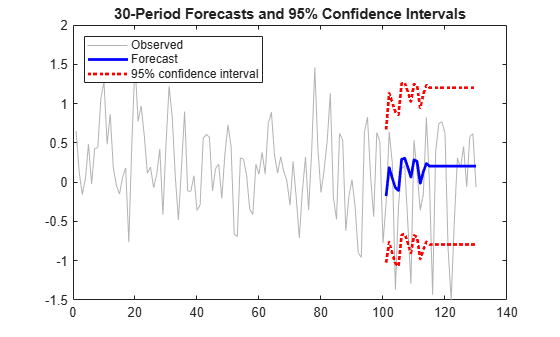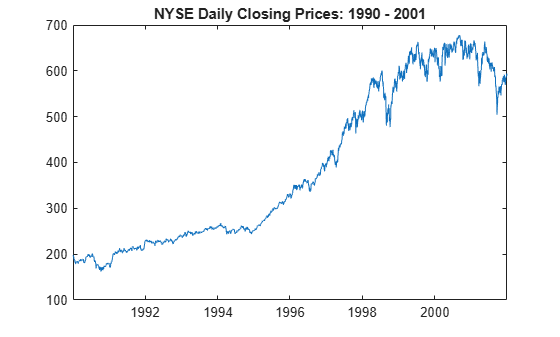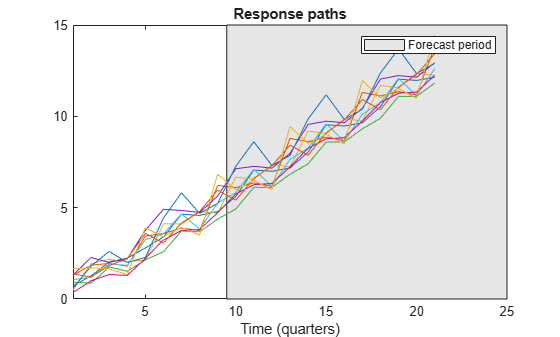# forecast

Forecast ARIMA or ARIMAX model responses or conditional variances

## Syntax

```[Y,YMSE] = forecast(Mdl,numperiods,Y0) [Y,YMSE] = forecast(Mdl,numperiods,Y0,Name,Value) [Y,YMSE,V] = forecast(___) ```

## Description

```[Y,YMSE] = forecast(Mdl,numperiods,Y0)``` returns `numperiods` consecutive forecasted responses `Y` and corresponding mean square errors `YMSE` of the fully specified, univariate ARIMA or ARIMAX model `Mdl`. The presample response data `Y0` initializes the model to generate forecasts.

`[Y,YMSE] = forecast(Mdl,numperiods,Y0,Name,Value)` uses additional options specified by one or more name-value pair arguments. For example, for a model with a regression component, `'X0',X0,'XF',XF` specifies the presample and forecasted predictor data `X0` and `XF`, respectively.

```[Y,YMSE,V] = forecast(___)``` also forecasts `numperiods` conditional variances `V` of a composite conditional mean and variance model (for example, an ARIMA and GARCH composite model) using any of the input argument combinations in the previous syntaxes.

## Input Arguments

expand all

Fully specified ARIMA or ARIMAX model, specified as an `arima` model returned by `arima` or `estimate`.

The properties of `Mdl` cannot contain `NaN`s.

Forecast horizon, or the number of time points in the forecast period, specified as a positive integer.

Data Types: `double`

Presample response data used to initialize the model for forecasting, specified as a numeric column vector with length `numpreobs` or a `numpreobs`-by-`numpaths` numeric matrix.

Rows of `Y0` correspond to periods in the presample, and the last row contains the latest presample response. `numpreobs` is the number of specified presample responses and it must be at least `Mdl.P`. If `numpreobs` exceeds `Mdl.P`, `forecast` uses only the latest `Mdl.P` rows. For more details, see Time Base Partitions for Forecasting.

Columns of `Y0` correspond to separate, independent paths.

• If `Y0` is a column vector, `forecast` applies it to each forecasted path. In this case, all forecast paths `Y` derive from the same initial conditions.

• If `Y0` is a matrix, it must have `numpaths` columns, where `numpaths` is the maximum among the second dimensions of the specified presample observation arrays `Y0`, `E0`, and `V0`.

Data Types: `double`

### Name-Value Pair Arguments

Specify optional comma-separated pairs of `Name,Value` arguments. `Name` is the argument name and `Value` is the corresponding value. `Name` must appear inside quotes. You can specify several name and value pair arguments in any order as `Name1,Value1,...,NameN,ValueN`.

Presample innovations used to initialize the moving average (MA) component of the model or conditional variance model, specified as the comma-separated pair consisting of `'E0'` and a numeric column vector or a numeric matrix with `numpaths` columns. `forecast` assumes that the presample innovations have a mean of 0.

Rows of `E0` correspond to periods in the presample, and the last row contains the latest presample innovation. `E0` must have at least `Mdl.Q` rows to initialize the MA component. If `Mdl.Variance` is a conditional variance model (for example, `garch`), `E0` might require more than `Mdl.Q` rows. If the number of rows exceeds the minimum number required to forecast `Mdl`, `forecast` uses only the latest required rows.

Columns of `E0` correspond to separate, independent paths.

• If `E0` is a column vector, `forecast` applies it to each forecasted path. In this case, the MA component and conditional variance model of all forecast paths `Y` derive from the same initial innovations.

• If `E0` is a matrix, it must have `numpaths` columns.

• By default, if `numpreobs``Mdl.P` + `Mdl.Q`, `forecast` infers any necessary presample innovations by passing the model `Mdl` and presample data to `infer`. For more details on this default for models containing a regression component, see `X0` and `XF`.

• By default, if `numpreobs` < `Mdl.P` + `Mdl.Q`, `forecast` sets all necessary presample innovations to `0`.

Data Types: `double`

Presample conditional variances used to initialize the conditional variance model, specified as the comma-separated pair consisting of `'V0'` and a positive numeric column vector or a positive numeric matrix with `numpaths` columns. If the model variance `Mdl.Variance` is constant, `forecast` ignores `V0`.

Rows of `V0` correspond to periods in the presample, and the last row contains the latest presample conditional variance. If `Mdl.Variance` is a conditional variance model (for example, a `garch` model object), `E0` might require more than `Mdl.Q` rows to initialize `Mdl` for forecasting. If the number of rows exceeds the minimum number required to forecast, the `forecast` function uses only the latest required presample conditional variances.

Columns of `V0` correspond to separate, independent paths.

• If `V0` is a column vector, `forecast` applies it to each forecasted path. In this case, the conditional variance model of all forecast paths `Y` derive from the same initial conditional variances.

• If `V0` is a matrix, it must have `numpaths` columns.

• By default, if you specify enough presample innovations `E0` to initialize the conditional variance model, `forecast` infers any necessary presample conditional variances by passing the conditional variance model `Mdl.Variance` and `E0` to `infer`.

• By default, if you do not specify `E0`, but you specify enough presample responses `Y0` to infer enough presample innovations, then `forecast` infers any necessary presample conditional variances from the inferred presample innovations.

• By default, if you do not specify enough presample data, `forecast` sets all necessary presample conditional variances to the unconditional variance of the variance process.

Data Types: `double`

Presample predictor data used to infer presample innovations `E0`, specified as the comma-separated pair consisting of `'X0'` and a numeric matrix with `numpreds` columns.

Rows of `X0` correspond to periods in the presample, and the last row contains the latest set of presample predictor observations.

• If you do not specify `E0`, `X0` must have at least `numpreobs``Mdl.P` rows so that `forecast` can infer presample innovations. If the number of rows exceeds the minimum number required to infer presample innovations, `forecast` uses only the latest required presample predictor observations. A best practice is to set `X0` to the same predictor data matrix used in the estimation, simulation, or inference of `Mdl`. This setting ensures the correct estimation of the presample innovations `E0`.

• If you specify `E0`, then `forecast` ignores `X0`.

Columns of `X0` represent separate time series variables, and they correspond to the columns of `XF`.

If you specify `X0` but you do not specify forecasted predictor data `XF`, then `forecast` issues an error.

By default, `forecast` drops the regression component from the model when it infers presample innovations, regardless of the value of the regression coefficient `Mdl.Beta`.

Data Types: `double`

Forecasted or future predictor data, specified as the comma-separated pair consisting of `'XF'` and a numeric matrix with `numpreds` columns. `XF` represents the evolution of specified presample predictor data `X0` forecasted into the future (the forecast period).

Rows of `XF` correspond to time points in the future; `XF(t,:)` contains the `t`-period-ahead predictor forecasts. `XF` must have at least `numperiods` rows. If the number of rows exceeds `numperiods`, `forecast` uses only the first `numperiods` forecasts. For more details, see Time Base Partitions for Forecasting.

Columns of `XF` are separate time series variables, and they correspond to the columns of `X0`.

By default, `forecast` generates forecasts from `Mdl` without a regression component, regardless of the value of the regression coefficient `Mdl.Beta`.

### Notes

`forecast` assumes that you synchronize all specified presample data sets such that the latest observation of each presample series occurs simultaneously. Similarly, `forecast` assumes that the first observation in the forecasted predictor data `XF` occurs in the next time point after the last observation in the presample predictor data `X0`.

## Output Arguments

expand all

Minimum mean square error (MMSE) forecasts of the conditional mean of the response data, returned as a `numperiods`-by-`numpaths` numeric matrix. `Y` represents a continuation of `Y0` (`Y(1,:)` occurs in the next time point after `Y0(end,:)`).

`Y(t,:)` contains the conditional mean forecast of all paths for time point `t` in the forecast period (the `t`-period-ahead forecasts).

`forecast` determines `numpaths` from the number of columns in the presample data sets `Y0`, `E0`, and `V0`. For details, see Algorithms. If each presample data set has one column, then `Y` is a column vector.

Data Types: `double`

Mean square errors (MSEs) of the forecasted responses `Y` (or forecast error variances), returned as a `numperiods`-by-`numpaths` numeric matrix.

`YMSE(t,:)` contains the forecast error variances of all paths for time point `t` in the forecast period.

`forecast` determines `numpaths` from the number of columns in the presample data sets `Y0`, `E0`, and `V0`. For details, see Algorithms. If you do not specify any presample data sets or each data set is a column vector, then `YMSE` is a column vector.

The square roots of `YMSE` are the standard errors of the forecasts `Y`.

Data Types: `double`

Minimum mean square error (MMSE) forecasts of the conditional variances of future model innovations, returned as a `numperiods`-by-`numpaths` numeric matrix. `V` has `numPeriods` rows and `numPaths` columns.

`forecast` sets the number of columns of `V` (`numPaths`) to the largest number of columns in the presample arrays `Y0`, `E0`, and `V0`. If you do not specify `Y0`, `E0`, and `V0`, then `V` is a `numPeriods` column vector.

In all cases, row i contains the conditional variance forecasts for the ith period.

Data Types: `double`

## Examples

expand all

Forecast the conditional mean response of simulated data over a 30-period horizon.

Simulate 130 observations from a multiplicative seasonal MA model with known parameter values.

```Mdl = arima('MA',{0.5,-0.3},'SMA',0.4,'SMALags',12,... 'Constant',0.04,'Variance',0.2); rng(200); Y = simulate(Mdl,130);```

Fit a seasonal MA model to the first 100 observations, and reserve the remaining 30 observations to evaluate forecast performance.

```MdlTemplate = arima('MALags',1:2,'SMALags',12); EstMdl = estimate(MdlTemplate,Y(1:100));```
``` ARIMA(0,0,2) Model with Seasonal MA(12) (Gaussian Distribution): Value StandardError TStatistic PValue ________ _____________ __________ __________ Constant 0.20403 0.069064 2.9542 0.0031344 MA{1} 0.50212 0.097298 5.1606 2.4619e-07 MA{2} -0.20174 0.10447 -1.9312 0.053464 SMA{12} 0.27028 0.10907 2.478 0.013211 Variance 0.18681 0.032732 5.7073 1.148e-08 ```

`EstMdl` is a new `arima` model that contains estimated parameters (that is, a fully specified model).

Forecast the fitted model into a 30-period horizon. Specify the estimation period data as a presample.

```[YF,YMSE] = forecast(EstMdl,30,Y(1:100)); YF(15)```
```ans = 0.2040 ```
`YMSE(15)`
```ans = 0.2592 ```

`YF` and `YMSE` are 30-by-1 vectors of forecasted responses and corresponding MSEs, respectively. The 15-period-ahead forecast is 0.2040 and its MSE is 0.2592.

Visually compare the forecasts to the holdout data.

```figure h1 = plot(Y,'Color',[.7,.7,.7]); hold on h2 = plot(101:130,YF,'b','LineWidth',2); h3 = plot(101:130,YF + 1.96*sqrt(YMSE),'r:',... 'LineWidth',2); plot(101:130,YF - 1.96*sqrt(YMSE),'r:','LineWidth',2); legend([h1 h2 h3],'Observed','Forecast',... '95% Confidence Interval','Location','NorthWest'); title(['30-Period Forecasts and Approximate 95% '... 'Confidence Intervals']) hold off```Forecast the daily NASDAQ Composite Index over a 500-day horizon.

Load the NASDAQ data included with the toolbox, and extract the first 1500 observations.

```load Data_EquityIdx nasdaq = DataTable.NASDAQ(1:1500);```

Fit an ARIMA(1,1,1) model to the data.

```nasdaqModel = arima(1,1,1); nasdaqFit = estimate(nasdaqModel,nasdaq);```
``` ARIMA(1,1,1) Model (Gaussian Distribution): Value StandardError TStatistic PValue _________ _____________ __________ __________ Constant 0.4303 0.18555 2.319 0.020396 AR{1} -0.074386 0.081985 -0.90731 0.36424 MA{1} 0.31125 0.077266 4.0283 5.6175e-05 Variance 27.826 0.63625 43.735 0 ```

Forecast the Composite Index for 500 days using the fitted model. Use the observed data as presample data.

`[Y,YMSE] = forecast(nasdaqFit,500,nasdaq);`

Plot the forecasts and 95% forecast intervals.

```lower = Y - 1.96*sqrt(YMSE); upper = Y + 1.96*sqrt(YMSE); figure plot(nasdaq,'Color',[.7,.7,.7]); hold on h1 = plot(1501:2000,lower,'r:','LineWidth',2); plot(1501:2000,upper,'r:','LineWidth',2) h2 = plot(1501:2000,Y,'k','LineWidth',2); legend([h1 h2],'95% Interval','Forecast',... 'Location','NorthWest') title('NASDAQ Composite Index Forecast') hold off```The process is nonstationary, so the widths of the forecast intervals grow with time.

Forecast the following known ARX(1) model into a 10-period forecast horizon:

`${y}_{t}=1+0.3{y}_{t-1}+2{x}_{t}+{\epsilon }_{t},$`

where ${\epsilon }_{\mathit{t}}$ is a standard Gaussian random variable, and ${\mathit{x}}_{\mathit{t}}$ is an exogenous Gaussian random variable with a mean of 1 and a standard deviation of 0.5.

Create an `arima` model object that represents the ARX(1) model.

`Mdl = arima('Constant',1,'AR',0.3,'Beta',2,'Variance',1);`

To forecast responses from the ARX(1) model, `forecast` requires:

• One presample response ${\mathit{y}}_{0}$ to initialize the autoregressive term

• Future exogenous data to include the effects of the exogenous variable on the forecasted responses

Set the presample response to the unconditional mean of the stationary process:

`$E\left({y}_{t}\right)=\frac{1+2\left(1\right)}{1-0.3}.$`

For the future exogenous data, draw 10 values from the distribution of the exogenous variable.

```rng(1); y0 = (1 + 2)/(1 - 0.3); xf = 1 + 0.5*randn(10,1);```

Forecast the ARX(1) model into a 10-period forecast horizon. Specify the presample response and future exogenous data.

```fh = 10; yf = forecast(Mdl,fh,y0,'XF',xf)```
```yf = 10×1 3.6367 5.2722 3.8232 3.0373 3.0657 3.3470 3.4454 4.2120 4.0667 4.8065 ```

`yf(3)` = `3.8232` is the 3-period-ahead forecast of the ARX(1) model.

Forecast multiple response paths from a known SAR$\left(1,0,0\right){\left(1,1,0\right)}_{4}$ model by specifying multiple presample response paths.

Create an `arima` model object that represents this quarterly SAR$\left(1,0,0\right){\left(1,1,0\right)}_{4}$ model:

`$\left(1-0.5L\right)\left(1-0.2{L}^{4}\right)\left(1-{L}^{4}\right){y}_{t}=1+{\epsilon }_{t},$`

where ${\epsilon }_{\mathit{t}}$ is a standard Gaussian random variable.

```Mdl = arima('Constant',1,'AR',0.5,'Variance',1,... 'Seasonality',4,'SARLags',4,'SAR',0.2)```
```Mdl = arima with properties: Description: "ARIMA(1,0,0) Model Seasonally Integrated with Seasonal AR(4) (Gaussian Distribution)" Distribution: Name = "Gaussian" P: 9 D: 0 Q: 0 Constant: 1 AR: {0.5} at lag  SAR: {0.2} at lag  MA: {} SMA: {} Seasonality: 4 Beta: [1×0] Variance: 1 ```

Because `Mdl` contains autoregressive dynamic terms, `forecast` requires the previous `Mdl.P` responses to generate a $\mathit{t}$-period-ahead forecast from the model. Therefore, the presample should contain nine values.

Generate a random 9-by-10 matrix representing 10 presample paths of length 9.

```rng(1); numpaths = 10; Y0 = rand(Mdl.P,numpaths);```

Forecast 10 paths from the SAR model into a 12-quarter forecast horizon. Specify the presample observation paths `Y0`.

```fh = 12; YF = forecast(Mdl,fh,Y0);```

`YF` is a 12-by-10 matrix of independent forecasted paths. `YF(j,k)` is the `j`-period-ahead forecast of path `k`. Path `YF(:,k)` represents the continuation of the presample path `Y0(:,k)`.

Plot the presample and forecasts.

```Y = [Y0;... YF]; figure; plot(Y); hold on h = gca; px = [6.5 h.XLim([2 2]) 6.5]; py = h.YLim([1 1 2 2]); hp = patch(px,py,[0.9 0.9 0.9]); uistack(hp,"bottom"); axis tight legend("Forecast period") xlabel('Time (quarters)') ylabel('Response paths')```expand all

## Algorithms

• `forecast` sets the number of sample paths to forecast `numpaths` to the maximum number of columns among the presample data sets `E0`, `V0`, and `Y0`. All presample data sets must have either `numpaths` > 1 columns or one column. Otherwise, `forecast` issues an error. For example, if `Y0` has five columns, representing five paths, then `E0` and `V0` can either have five columns or one column. If `E0` has one column, then `forecast` applies `E0` to each path.

• `NaN` values in presample and future data sets indicate missing data. `forecast` removes missing data from the presample data sets following this procedure:

1. `forecast` horizontally concatenates the specified presample data sets `Y0`, `E0`, `V0`, and `X0` such that the latest observations occur simultaneously. The result can be a jagged array because the presample data sets can have a different number of rows. In this case, `forecast` prepads variables with an appropriate amount of zeros to form a matrix.

2. `forecast` applies list-wise deletion to the combined presample matrix by removing all rows containing at least one `NaN`.

3. `forecast` extracts the processed presample data sets from the result of step 2, and removes all prepadded zeros.

`forecast` applies a similar procedure to the forecasted predictor data `XF`. After `forecast` applies list-wise deletion to `XF`, the result must have at least `numperiods` rows. Otherwise, `forecast` issues an error.

List-wise deletion reduces the sample size and can create irregular time series.

• When `forecast` estimates MSEs `YMSE` of the conditional mean forecasts `Y`, the function treats the specified predictor data sets `X0` and `XF` as exogenous, nonstochastic, and statistically independent of the model innovations. Therefore, `YMSE` reflects the variance associated with the ARIMA component of the input model `Mdl` alone.

expand all

## References

 Baillie, R., and T. Bollerslev. “Prediction in Dynamic Models with Time-Dependent Conditional Variances.” Journal of Econometrics. Vol. 52, 1992, pp. 91–113.

 Bollerslev, T. “Generalized Autoregressive Conditional Heteroskedasticity.” Journal of Econometrics. Vol. 31, 1996, pp. 307–327.

 Bollerslev, T. “A Conditionally Heteroskedastic Time Series Model for Speculative Prices and Rates of Return.” The Review Economics and Statistics. Vol. 69, 1987, pp. 542–547.

 Box, G. E. P., G. M. Jenkins, and G. C. Reinsel. Time Series Analysis: Forecasting and Control 3rd ed. Englewood Cliffs, NJ: Prentice Hall, 1994.

 Enders, W. Applied Econometric Time Series. Hoboken, NJ: John Wiley & Sons, 1995.

 Engle, R. F. “Autoregressive Conditional Heteroskedasticity with Estimates of the Variance of United Kingdom Inflation.” Econometrica. Vol. 50, 1982, pp. 987–1007.

 Hamilton, J. D. Time Series Analysis. Princeton, NJ: Princeton University Press, 1994.